Nov 29, 2016

# Summarizing and Graphing Data — practice test 2A

36 cards
Directions:

• Provide an appropriate response.
• 1. Histograms and Pareto charts are both bar charts. What is the significant difference between the two?

(Answers will vary) Histograms convey quantitative information about shapes of distributions. Pareto charts convey comparative information about relative standing of categorical data.

1. 2. Suppose that a data set has a minimum value of 25 and a max of 75 and that you want 5 classes. Explain how to find the class width for this frequency table. What happens if you mistakenly use a class width of 10 instead of 11?

Since the range is 75 – 25 = 50, and 50 divided by 5 equals 10, a whole number, the class width has to be widened from 10 to 11. In that way, data values equal to 75 will not be omitted from the frequency table.

2. Solve the problem.
• 3. Using the employment information in the table on Alpha Corporation, determine the width of each class.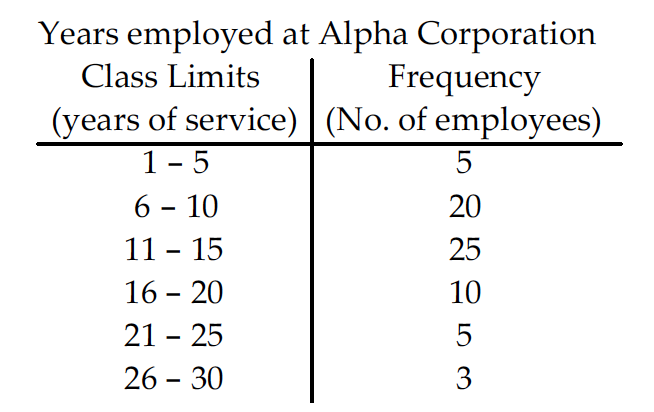1. 5
2. 4
3. 6
4. 10

1. 4. Using the information in the table on home sale prices in the city of Summerhill for the month of June, find the class boundaries for class 80.0 – 110.9.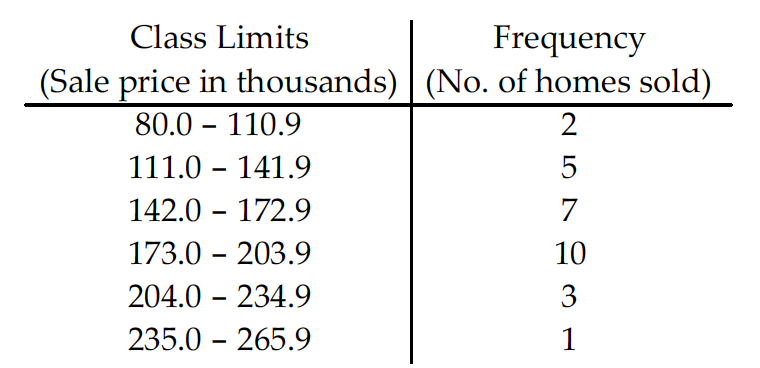1. 80.00, 110.95
2. 79.90, 110.95
3. 79.90, 110.0
4. 79.95, 110.95

2. Construct the relative frequency distribution that corresponds to the given frequency distribution.
• 5.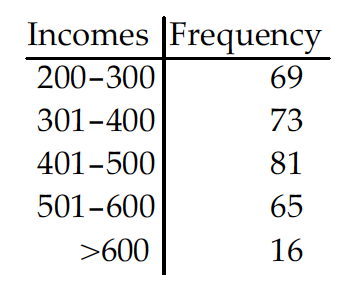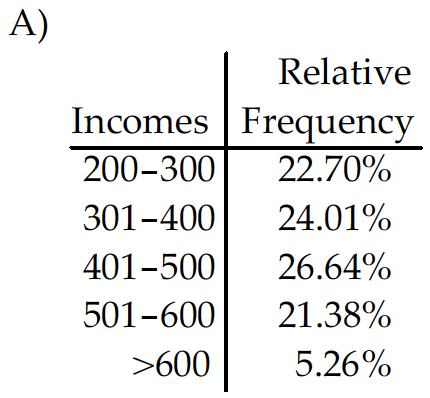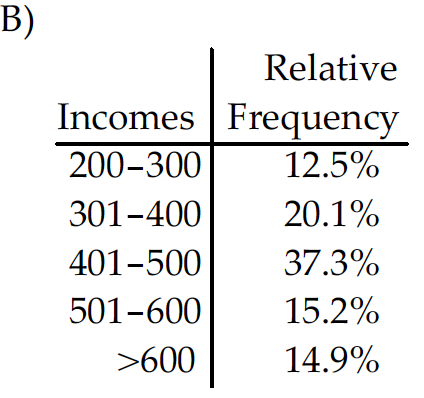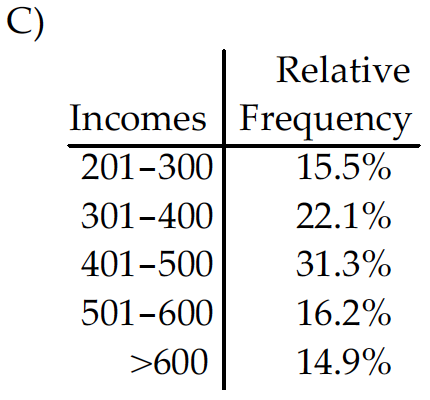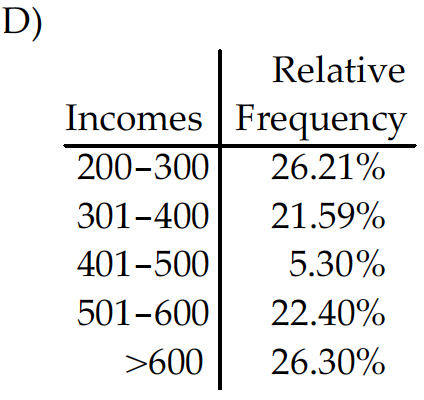1. the relative frequency distribution D
2. the relative frequency distribution B
3. the relative frequency distribution A
4. the relative frequency distribution C

• Solve the problem.
• 6. Use the high closing values of Naristar Inc. stock from the years 1990 – 2001 to construct a time-series graph. (Let x = 0 stand for 1990 and so on…) Identify a trend.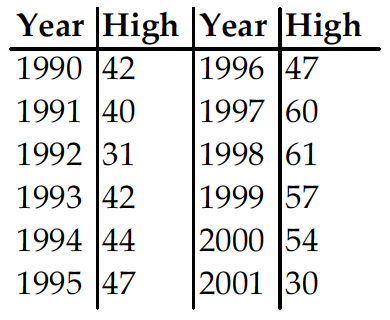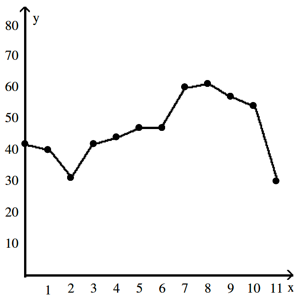Trend: (Answers will vary) High closing stock values show a decrease from 1990 through 1992, after which the value of the stock rose through 1998. Another decrease occurred in 1999 and continued through 2001.

2. Construct the cumulative frequency distribution that corresponds to the given frequency distribution.
• 7.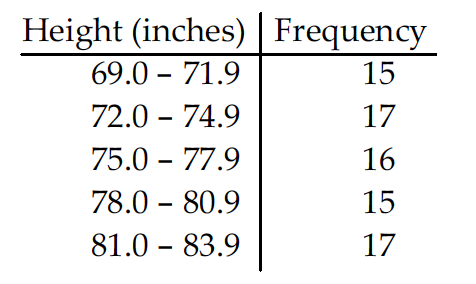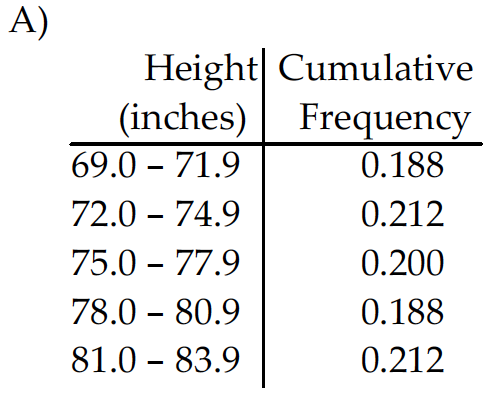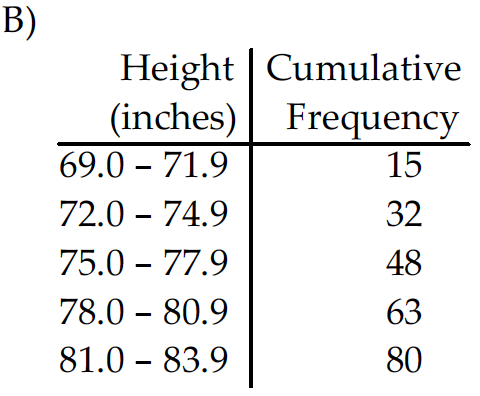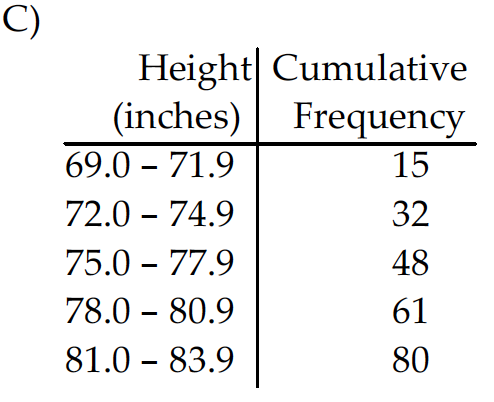1. the cumulative frequency distribution B
2. the cumulative frequency distribution D
3. the cumulative frequency distribution A
4. the cumulative frequency distribution C

• Provide an appropriate response.
• 8. Sturges’ guideline suggests that when constructing a frequency distribution, the ideal number of classes can be approximated by 1+(log n)/(log 2), where n is the number of data values. Use this guideline to find the ideal number of classes when the number of data values is 50. Round your answer to the nearest whole number.

A nurse measured the blood pressure of each person who visited her clinic. Following is a relative-frequency histogram for the systolic blood pressure readings for those people aged between 25 and 40. Use the histogram to answer the question. The blood pressure readings were given to the nearest whole number.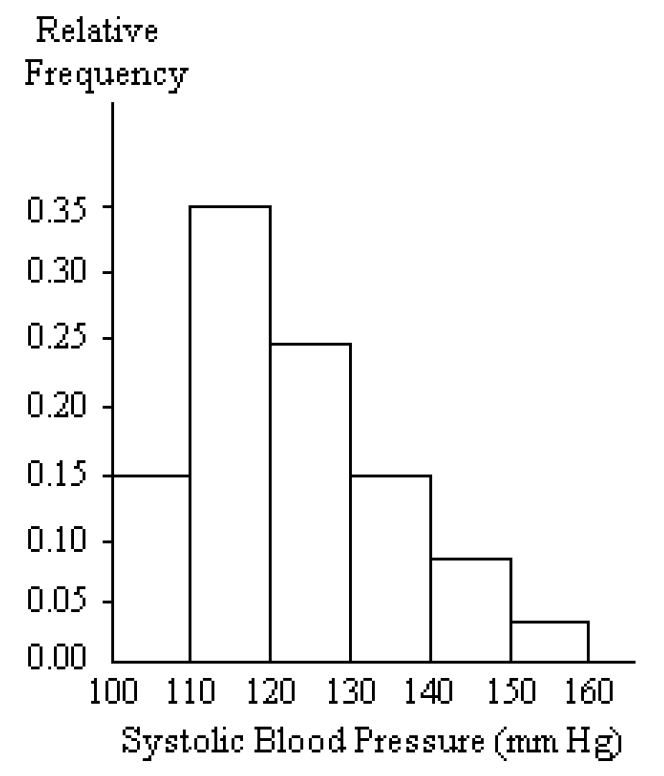1. 9
2. 7
3. 6
4. 8

1. 9. Approximately what percentage of the people aged 25–40 had a systolic blood pressure reading between 110 and 119 inclusive?
1. 30%
2. 0.35%
3. 35%
4. 3.5%

• Provide and appropriate response.
• 10. Suppose that a histogram is constructed for the frequency distribution shown below: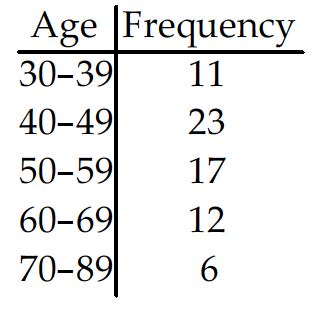The class 60–69 has twice the frequency of the class 70–89. In the histogram, will the area of the bar for the class 60–69 be twice the area of the bar for the class 70-89? In other words, will areas be proportional to frequencies in this histogram? Explain your thinking. Are there any conditions under which areas are proportional to frequencies in histograms?

The areas of the bars for the two classes will actually be the same. This is because the bar for the class 60-69, while it is twice as tall as the bar for the class 70-89, is also only half the width because the class widths are not the same. Heights, not areas, are proportional to frequencies. For classes of equal width, areas will also be proportional to frequencies.

2. Use the pie chart to solve the problem.
• 11. A survey of the 9225 vehicles on the campus of State University yielded the following circle graph.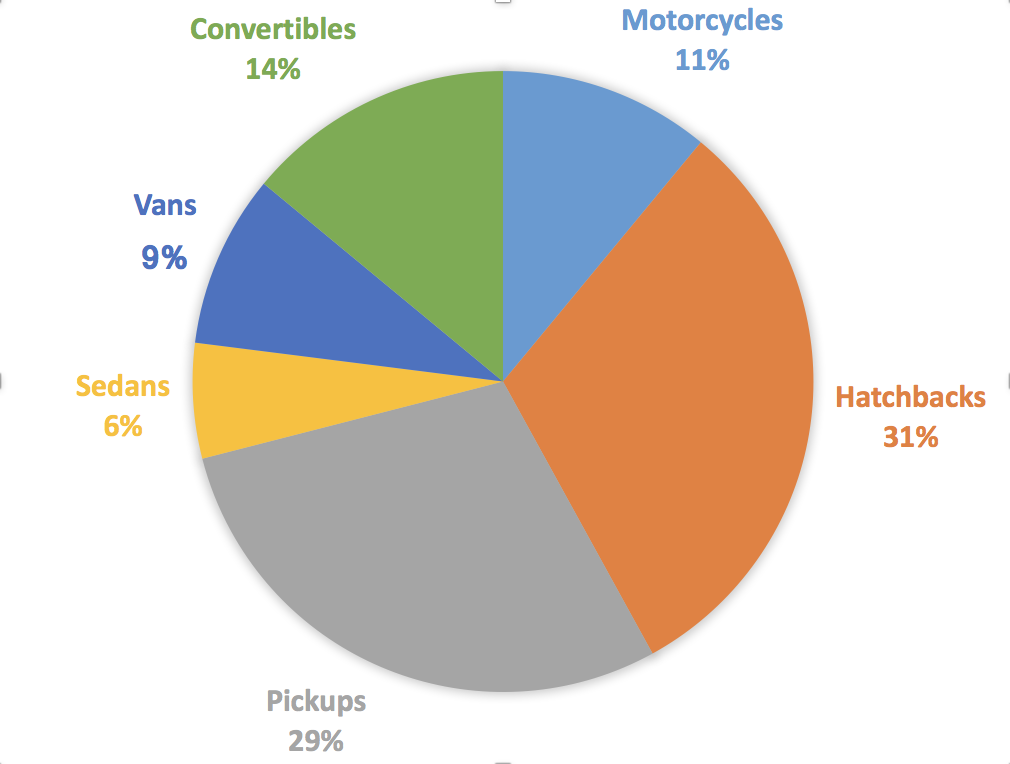1. Find the number of hatchbacks. Round your result to the nearest whole number.
1. 286
2. 2860
3. 2675
4. 268

2. 12. The pie chart below gives the inventory of the men’s department of a store.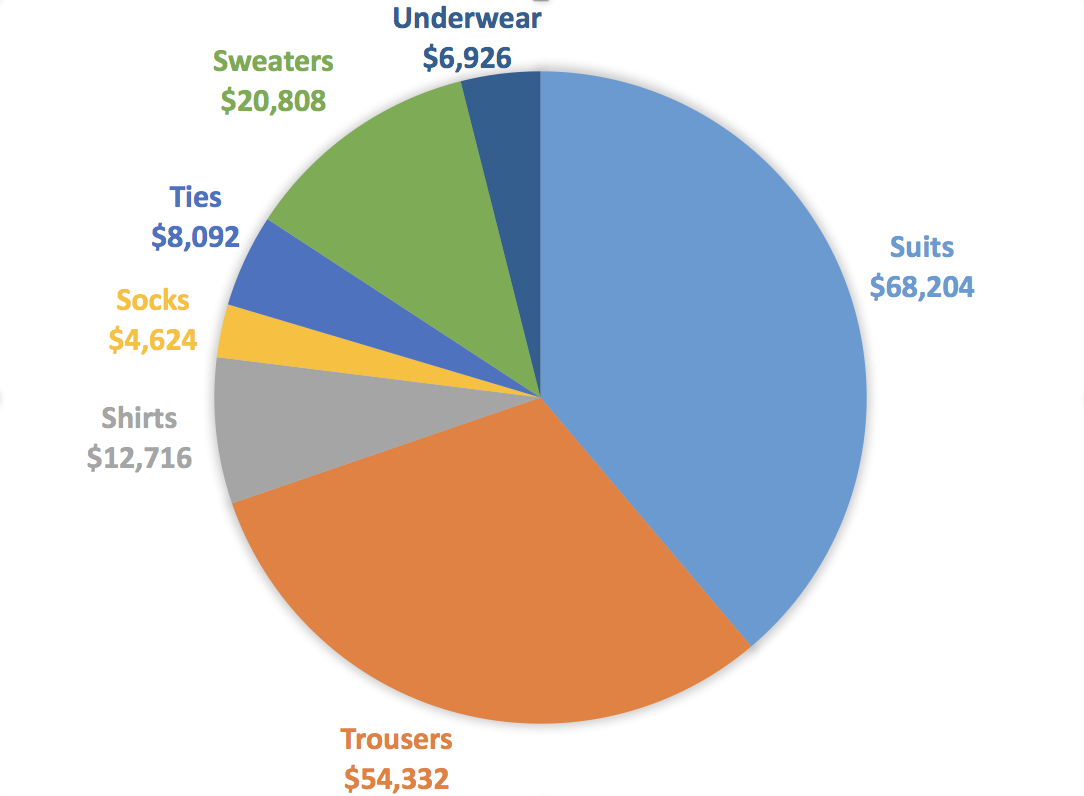1. What is the total inventory?
1. \$175,712
2. \$180,336
3. \$171,088
4. \$121,380

3. Find the original data from the stem-and-leaf plot.
• 13.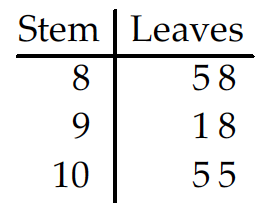1. 85, 81, 88, 91, 101, 105
2. 85, 88, 91, 91, 105, 105
3. 81, 85, 81, 98, 108, 105
4. 85, 88, 91, 98, 105, 105

1. 14.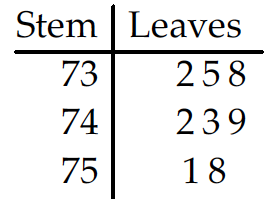1. 732, 735, 738, 742, 743, 749, 751, 758
2. 75, 78, 81, 76, 77, 83, 76, 83
3. 732, 735, 748, 742, 743, 749, 751, 768
4. 73258, 74239, 7518

2. Construct the plot for the given data.
• 15. Create the dot plot: A manufacturer records the number of errors each work station makes during the week. The data are as follows:
6 3 2 3 5 2 0 2 5 4 2 0 1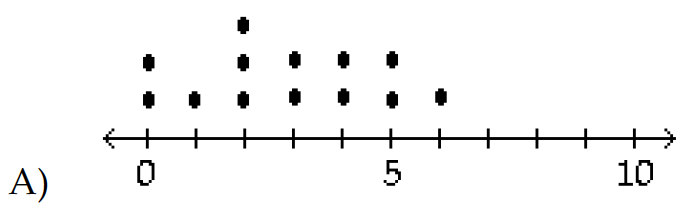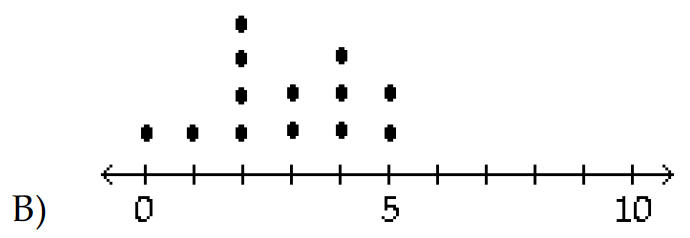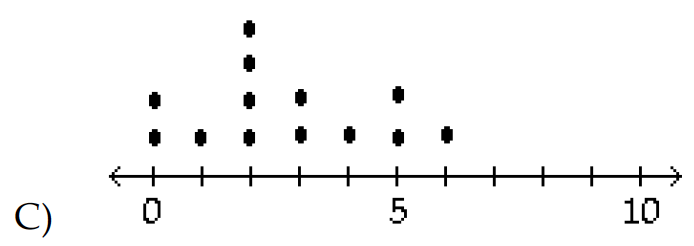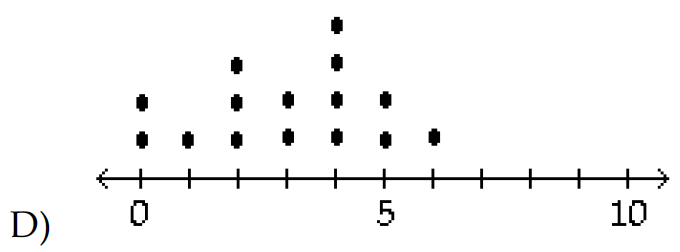1. dot plot C
2. dot plot D
3. dot plot A
4. dot plot B

• Use the data to create a stemplot.
• 16. The midterm test scores for the seventh-period typing class are listed below.
85 77 93 91 74 65 68 97 88 59 74 83 85 72 63 79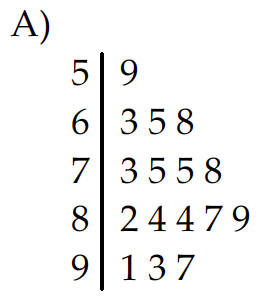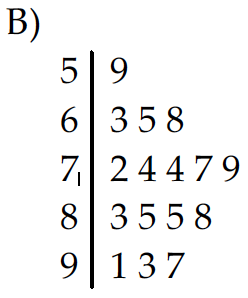1. Stemplot A
2. Stemplot B

• Use the given paired data to construct a scatterplot.
• 17.
x 2 -1 -1 -6 3 3 1 8 -5 -1
y 3 -3 -1 -9 2 -1 -4 2 -3 -2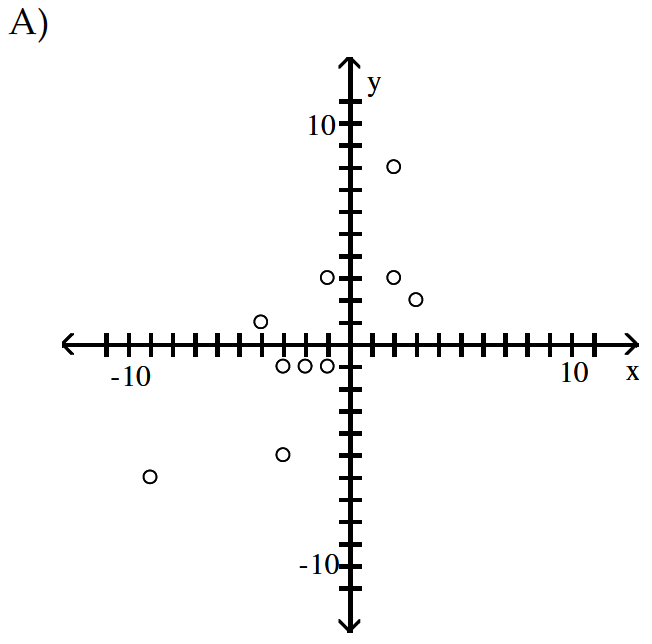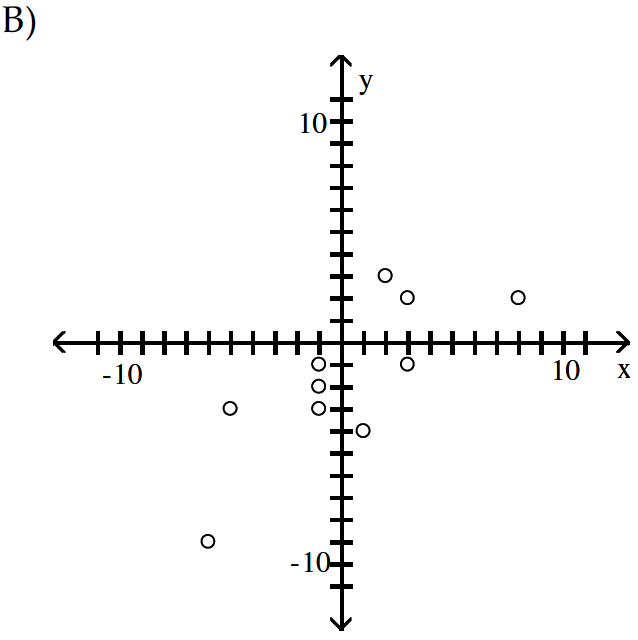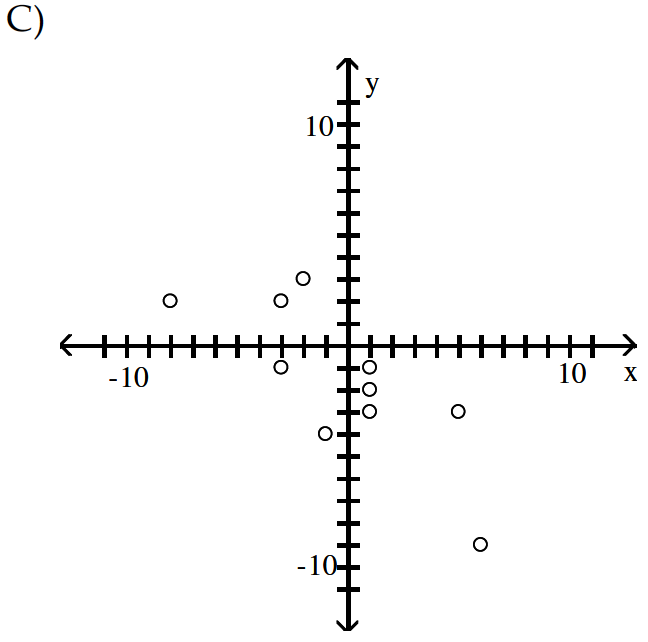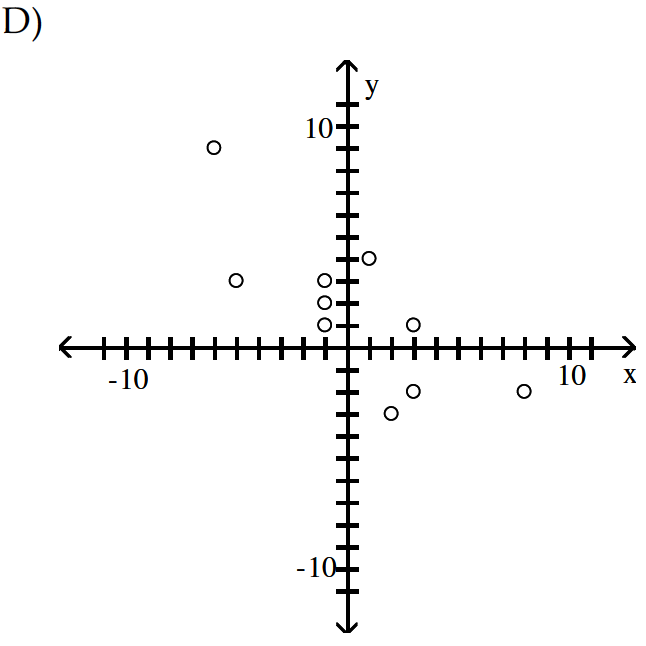1. Scatterplot B
2. Scatterplot D
3. Scatterplot A
4. Scatterplot C

• Choose the vertical scale and construct a Pareto chart.
• 18. A car dealer is deciding what kinds of vehicles he should order from the factory. He looks at his sales report for the preceding period. Choose the vertical scale so that the relative frequencies are represented.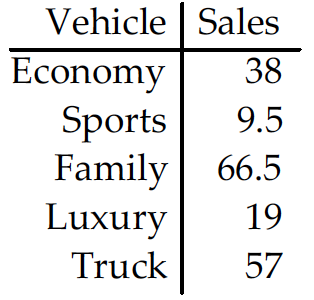Construct a Pareto chart to help him decide.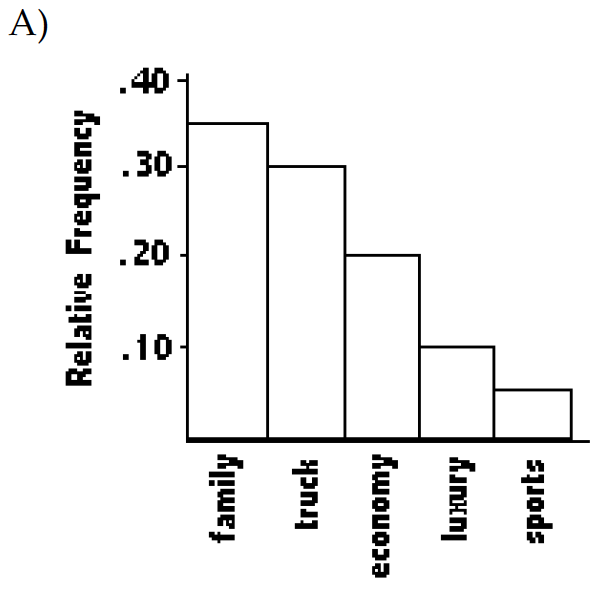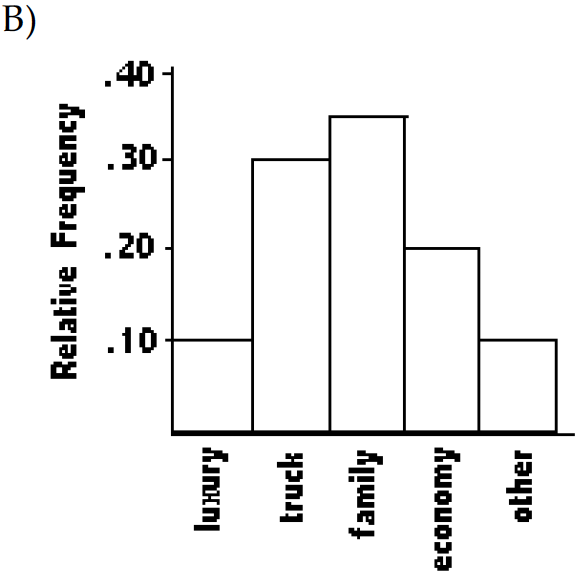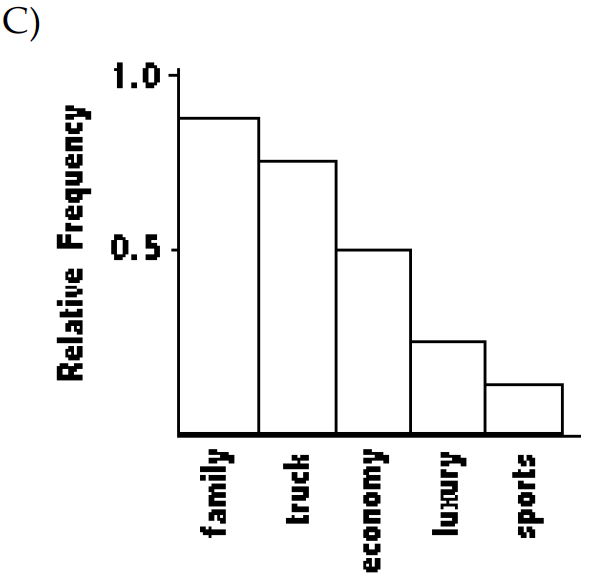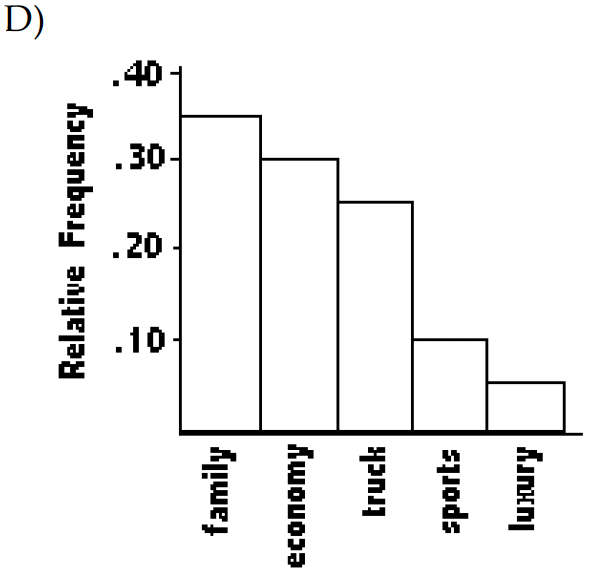1. Pareto chart B
2. Pareto chart C
3. Pareto chart D
4. Pareto chart A

• Construct a pie chart representing the given data set.
• 19. The following data dive the distribution of the types of houses in a town containing 18,000 houses.

Capes — 4500
Garrisons — 6300
Splits — 7200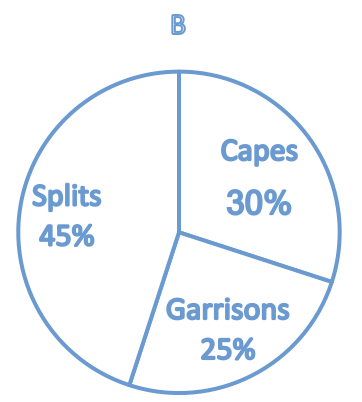1. Pie chart A
2. Pie chart B

• Provide an appropriate response.
• 20. One purpose of displaying data graphically is to provide clues about trends. The given values are weights (ounces) of steaks listed on a restaurant menu as “20-ounce porterhouse” steaks. The weights are supposed to be 21 ounces because they supposedly lose an ounce when cooked. Create a frequency distribution with 5 classes. Based on your distribution, comment on the advertised “20 ounces” steaks.
17 20 21 18 20 20 20 18 19 19 20 19 21 20 18 20 20 19 18 19